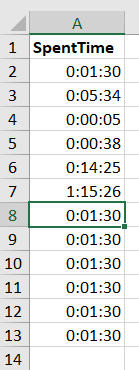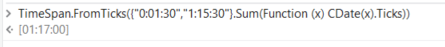# Sum timeHello, I have a column in excel that is in time format. how can I get the sum of all rows? total time

give a try at

Assign Activity:
AllTimes | DataType: TimeSpan =
TimeSpan.FromTicks(YourDTVar.AsEnumerable.Sum(Function (x) CDate(x(“SpentTime”).toString.Trim).Ticks))

Proto:1 Like

Hi

variable time is GenericValue, we need to convert that to TimeSpan type and then use .Add() method to add the time

more details

after Format value activity

• create a variable of System.TimeSpan type, lets say TempTime
• assign TempTime = TimeSpan.Parse(time) this converts the GenericValue of time to TimeSpan type
• create another variable lets say total_time of TimeSpan type, assign value 0 before the For Each Row. Now within the for loop assing total_time = total_time.Add(TempTime) this will add all the times in the excel

Regards,
Dhanush